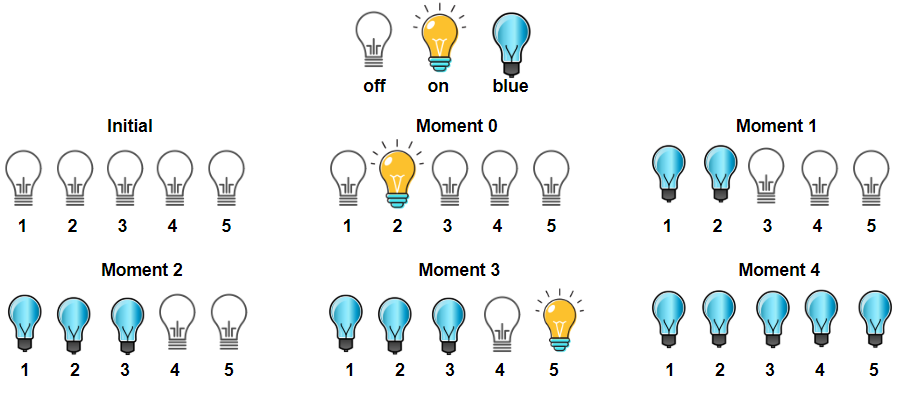Minos Park

Bulb Switcher III

February 2021

Problem #1375, LeetCode

There is a room with n bulbs, numbered from 1 to n, arranged in a row from left to right. Initially, all the bulbs are turned off.

At moment k (for k from 0 to n - 1), we turn on the light[k] bulb. A bulb change color to blue only if it is on and all the previous bulbs (to the left) are turned on too.

Return the number of moments in which all turned on bulbs are blue.

Example 1:Input: light = [2,1,3,5,4]
Output: 3
Explanation: All bulbs turned on, are blue at the moment 1, 2 and 4.

Example 2:

Input: light = [3,2,4,1,5]
Output: 2
Explanation: All bulbs turned on, are blue at the moment 3, and 4 (index-0).

Example 3:

Input: light = [4,1,2,3]
Output: 1
Explanation: All bulbs turned on, are blue at the moment 3 (index-0).
Bulb 4th changes to blue at the moment 3.

Example 4:

Input: light = [2,1,4,3,6,5]
Output: 3

Example 5:

Input: light = [1,2,3,4,5,6]
Output: 6

Constraints:

n == light.length
1 <= n <= 5 * 10^4
light is a permutation of  [1, 2, ..., n]

Accepted 25,281 Submissions 39,421

Solution I

Note, given that at each moment we are turning a bulb on with no exception. Thus the following is true: for the bulbs are blue at the moment n, if and only if n lights are sequentially turned on from the left side.

This reduces the problem quite a bit, because we only need to keep track of how many bulbs are turned on left of the current index, as well as the index of the bulbs that are turned on right of the current index. We don’t need to keep track of left side, because the light array is a permutation – i.e. there are no repeating elements.

Code 184ms - 15% faster than other C++ submissions

class Solution {
public:
int numTimesAllBlue(vector<int>& light) {
// Note, light array values are based in 1-index
// we'll use 0-index
uint64_t index = 0;

// how many bulbs are on to the left side of index (inclusive)
uint64_t left = 0;

// Since we only track its presence
// for non-existent keys, the default should be false
// https://stackoverflow.com/questions/11058422/map-operator-and-bool-as-value
std::map<int, bool> right;

uint64_t blue = 0;

for (std::vector<int>::iterator it = light.begin(); it != light.end(); ++it) {
if (*it - 1<= index) {
left++;
}
else {
// keep track of index
right[*it-1] = true;
}

// Increase all blue moment count
// First, check if current index is in the right map
if (right[index]) {
right[index] = false;
left++;
}
if (index + 1 == left) {
blue++;
}

// increase current index
index++;
}
return blue;
}
};

Solution II

After considerations and viewing some discussions, I realized that the problem could be further reduced. The map in the above code could be eliminated, because the only data we need to keep track of is the most right bulb index that is on. For example, if a bulb is turned on in the right side of the index, then there will not be any moments where the lights are blue unless all the lights leading up to that bulb is turned on.

Code 48ms - 99.09% faster than other C++ submissions

class Solution {
public:
int numTimesAllBlue(vector<int>& light) {
// Note, light array values are based in 1-index
// we'll use 0-index
uint64_t index = 0;

// how many bulbs are on to the left side of index (inclusive)
uint64_t left = 0;

uint64_t blue = 0;

for (int i = 0; i < light.size(); i++) {
if (light[i] - 1> left) {
left = light[i] - 1;
}

// Increase all blue moment count
if (index == left) {
blue++;
}

// increase current index
index++;
}
return blue;
}
};

Tags: coding challenges